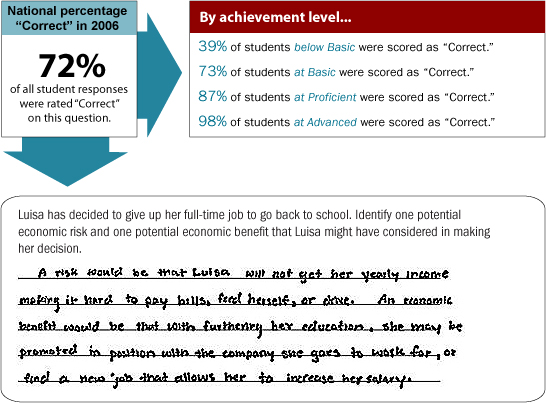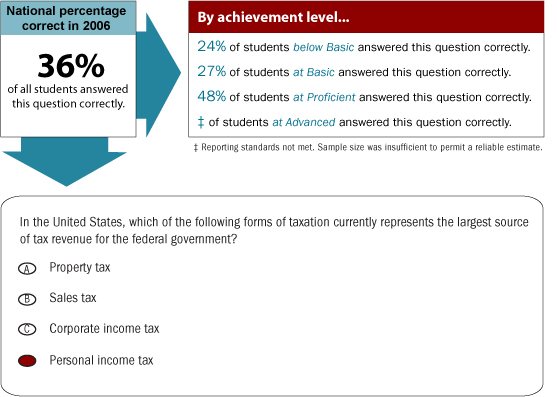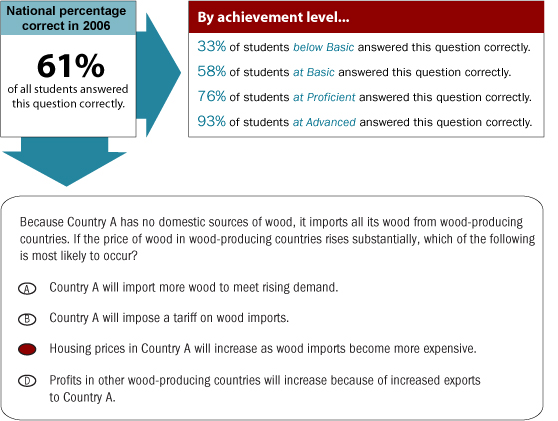...the official site for results from theNational Assessment of Educational ProgressHomeSample Questions in EconomicsThe constructed-response question on this page measured analysis and interpretation skills for the content area Market Economy. Student responses were scored according to a 3-point scale as "Correct," "Partially Correct," or "Incorrect." The sample response below is an example of a "Correct" response.

Overall, 72 percent of 12th-graders were scored as having a "Correct" response on this sample question. When only the students at the Proficient level are considered, 87 percent scored "Correct."View this question, at score 131, on a map of NAEP economics items.

Find out what the economics assessment measures.The following multiple-choice question from the grade 12 economics assessment measured student knowledge of the content area National Economy. The percentages below indicate how students performed on the question. In addition to the overall percentage of students who answered the question correctly, the percentage of students at each achievement level who answered the question correctly is presented.

As an example of how to interpret these percentages, 36 percent of the students overall answered this question correctly. When only the students at the Proficient level are considered, 48 percent answered correctly.View this question, at score 207, on a map of NAEP economics items.

Find out what the economics assessment measures.The following multiple-choice question from the grade 12 economics assessment measured student knowledge of the content area International Economy. The percentages below indicate how students performed on the question. In addition to the overall percentage of students who answered the question correctly, the percentage of students at each achievement level who answered the question correctly is presented.

As an example of how to interpret these percentages, 61 percent of the students overall answered this question correctly. When only the students at the Proficient level are considered, 76 percent answered correctly.